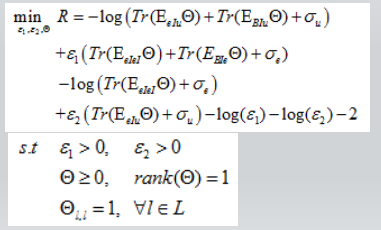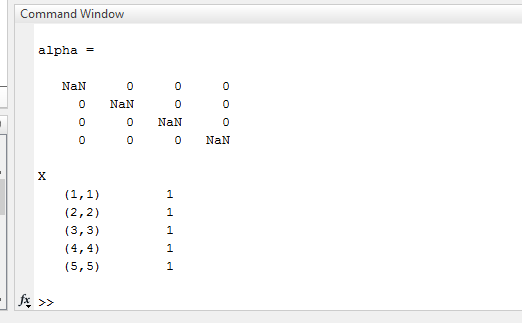# Why does the NAN answer come back after Gaussian Random?

Hi everyone.
I want to solve the following problem using CVX MATLAB , and since it is only one of the non-convex constraints, I used Gaussian Random for it, but in the end the answer we get is NAN.and my code is written as follows:
clc,close all,clear
ep1 =0.0086;
ep2 =0.0031;
var_u =10.^(-115/10);
var_e = var_u;
L=4;
S=zeros(L+1,L+1,L+1);
E_BuII=46.6770;
G_eIuu=14.37;
E_BeII=65.76;
g_eIeI=8.6689;
for i=1:L+1
S(i,i,i)=1;
end
n=L+1;
cvx_begin %sdp %quiet
%cvx_precision best
variable X(n,n) hermitian
minimize -(log(trace(XG_eIuu)+var_u+trace(XE_BuII))/log(2))-(log(trace(Xg_eIeI)+var_e)/log(2))+ep1(trace(XG_eIuu)+var_u)-(log(ep1)/log(2))+ep2(trace(Xg_eIeI)+var_e+trace(XE_BeII))-(log(ep2)/log(2))-2;
subject to
for i=1:L+1
(1e6*trace(S(:,:,i)*X))==1e6;
end
X == hermitian_semidefinite(L+1);
(X(n,n))>=0;
ep1>0;
ep2>0;
cvx_end
%% Gaussian randomization and x generation
[uu, cc]=eigs(X);
[a, b]=size(X);
x=sqrt(cc(b,b))*uu(:,b);
x=x./abs(x);
p=(x(1:L));
alpha=diag§
disp(X)I presume the solver failed, in some way, but you don’t show us the CVX and solver output, so I don’t know. <Edit: I no longer believe the sentence in this paragraph to be true - see my next post. But the remainder of this post is not withdrawn.>

I don’t know what `var_u` and `var_e` supposed to do, but having a number such as `1e-11.5` may cause numerical difficulties or unreliability in the solver.

Why are you multiplying both sides of a constraint by `1e6`? That is not a good thing to do.

I don’t know why you have the constraint `(X(n,n))>=0;`, but that only constrains one element of X`, namely, the n,n element of it. it does not, for instance, constrain all elements of the diagonal. And it is redundant because the semidefinite constraint forces all diagonal elements to be nonnegative.

Also, the strict inequality constraints will only be enforced as `>=`. If you need strict positivity, you’ll need to make the RHS something like 1e-5. Actually, your constraints on `ep` and `ep2` do nothing, because you set them to double precision values and did not declare them as variables, so those “constraints” will be evaluated by MATLAB (not CVX) as true, and do nothing in your optimization problem.

There’s probably plenty more wrong with your formulation ( i haven’t checked), so hopefully you will reexamine everything.

If you can use CvX 2.2 with Mosek 9.x as solver, use that. Otherwise, follow the advice at CVXQUAD: How to use CVXQUAD's Pade Approximant instead of CVX's unreliable Successive Approximation for GP mode, log, exp, entr, rel_entr, kl_div, log_det, det_rootn, exponential cone. CVXQUAD's Quantum (Matrix) Entropy & Matrix Log related functions

Re-reading your post, it looks like the CVX optimization problem was solved, with optimal value `X = eye(5)`, but in sparse form. I believe the first `L` elements of `x` are zero, which result in `NaN` when divided by their absolute value. Hence `p` is a 4 by 1 vector of `NaN`, and therefore `alpha` is a diagonal matrix with `NaN` values on the diagonal. These `NaN` values have nothing to do with CVX. They occurred in “straight” MATLAB due to dividing zero by zero in `x=x./abs(x);`

Given all the issues with your CVX program, some of which were described in my previous post, I would have no confidence that the identity matrix is the correct solution to the problem toy wanted to solve. As for `x=x./abs(x);`, I don’t know what the intended use is, but perhaps elements of `x` which have zero should remain at zero when it is zero prior to that statement. That would be the same as using MATLAB’s `sign` function, i.e., `x = sign(x)` instead of `x=x./abs(x)`.

Thank you very much for your help